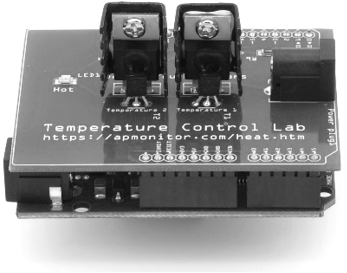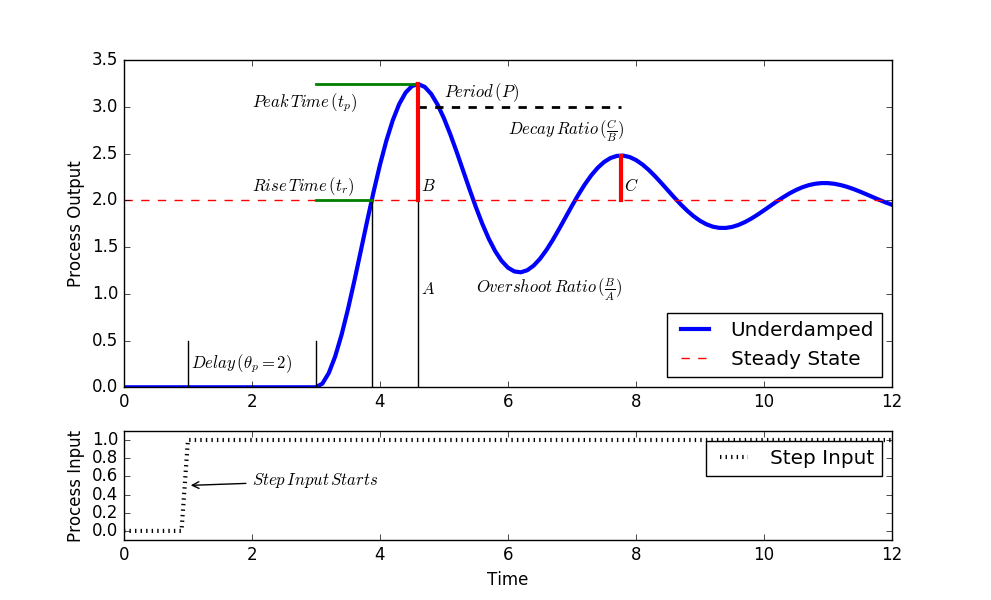## Quiz on Second Order Graphical Methods1. What type of second order system has oscillations?

A. Two first order systems
Incorrect. Two first order systems do not lead to oscillation but two first order systems in parallel may create an inverse response. Underdamped systems have an oscillatory response to a step change in the input.
B. Underdamped
Correct. Underdamped systems have an oscillatory response to a step change in the input
C. Overdamped
Incorrect. Underdamped systems have an oscillatory response to a step change in the input
D. Critically damped
Incorrect. Underdamped systems have an oscillatory response to a step change in the input

2. What is the relationship between the overshoot ratio (OS) and the decay ratio (DR)?

A. OS=DR
Incorrect. DR = OS^2 = \exp({-2 \pi \zeta}/{\sqrt{1-\zeta^2}})
B. OS=DR^2
Incorrect. DR = OS^2 = \exp({-2 \pi \zeta}/{\sqrt{1-\zeta^2}})
C.  DR=\sqrt{OS}
Incorrect. DR = OS^2 = \exp({-2 \pi \zeta}/{\sqrt{1-\zeta^2}})
D. DR=OS^2
Correct. DR = OS^2 = \exp({-2 \pi \zeta}/{\sqrt{1-\zeta^2}})

Information for Questions 3 and 4: An underdamped system has an Overshoot ratio (OS) of 0.5 and a peak time (t_p) of 0.2 min. The system is described by a second order equation.

$$\tau_s^2 \frac{d^2y}{dt^2} + 2 \zeta \tau_s \frac{dy}{dt} + y = K_p \, u\left(t-\theta_p \right)$$

Overshoot ratio OS: amount that first oscillation surpasses the steady state level relative to the steady state change

$$OS = \exp\left({-\frac{\pi \zeta}{\sqrt{1-\zeta^2}}}\right) \quad \quad \quad \zeta = \sqrt{\frac{\left(\ln(OS)\right)^2}{\pi^2 + \left(\ln(OS)\right)^2}}$$

Peak time t_p: amount of time to reach the first peak (after accounting for dead time)

$$t_p = \frac{\pi \tau_s}{\sqrt{1-\zeta^2}} \quad \quad \quad \tau_s = \frac{\sqrt{1-\zeta^2}}{\pi}t_p$$

Period P: the length of time for an oscillation from peak to peak

$$P = \frac{2 \pi \tau_s}{\sqrt{1-\zeta^2}} \quad \quad \tau_s = \frac{\sqrt{1-\zeta^2}}{2 \pi}P$$3. What is the value of \tau_s?

A. 0.0564
Incorrect. Square the \zeta in the equation for \tau_s
B. 0.0636
Incorrect. There is a square root in the equation for \zeta
C. 0.215
Incorrect. This is the value for \zeta.

$$\zeta = \sqrt{\frac{\left(\ln(0.5)\right)^2}{\pi^2 + \left(\ln(0.5)\right)^2}} = \sqrt{\frac{\left(\ln(0.5)\right)^2}{\pi^2 + \left(\ln(0.5)\right)^2}} = \sqrt{0.0464} = 0.215$$

D. 0.0622
Correct.

$$\zeta = \sqrt{\frac{\left(\ln(0.5)\right)^2}{\pi^2 + \left(\ln(0.5)\right)^2}} = \sqrt{\frac{\left(\ln(0.5)\right)^2}{\pi^2 + \left(\ln(0.5)\right)^2}} = \sqrt{0.0464} = 0.215$$

$$\tau_s = \frac{\sqrt{1-\zeta^2}}{\pi}t_p = \frac{\sqrt{1-0.215^2}}{\pi}0.2 = 0.0622$$

4. What is the period of oscillation P?

A. 0.4 min
Correct. P = {2 \pi \tau_s}/{\sqrt{1-\zeta^2}} = {2 \pi 0.0622}/{\sqrt{1-0.215^2}} = 0.4
B. 0.2 min
Incorrect. Use 2\pi on the top of the fraction
C. 0.44 min
Incorrect. Square \zeta
D. 0.4 sec
Incorrect. The units of time are from the value of t_p so seconds is incorrect unless there is a unit conversion to 24 sec = 0.4 min.